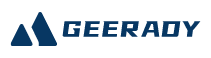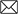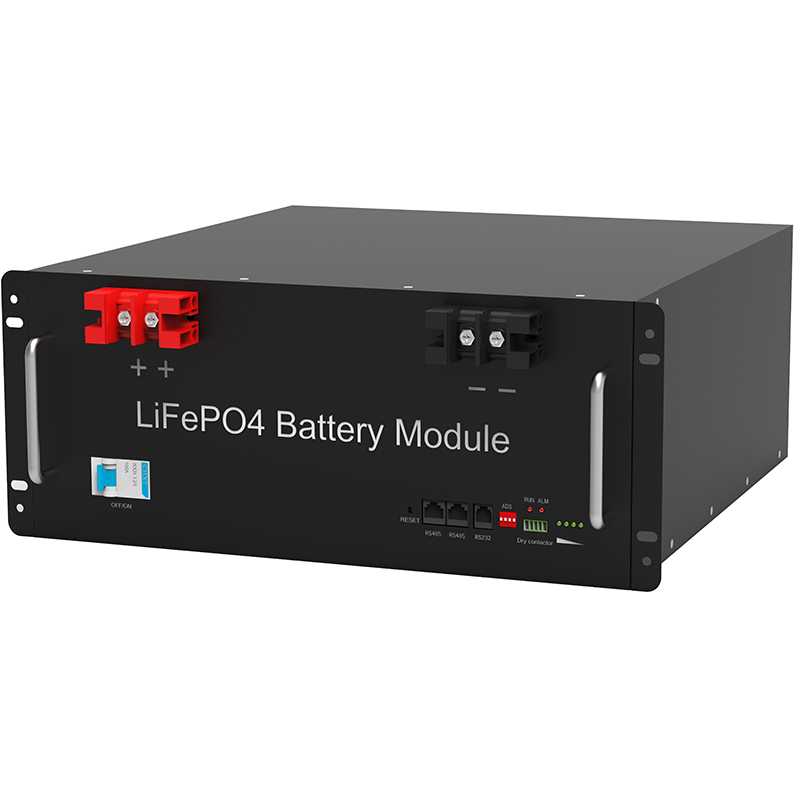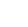#Emial:sales@geerady.com
Location： > > Differences of current tables and voltmeter use

### Differences of current tables and voltmeter use

Return Listsource：Gratene date： 2022-06-17Current tables and voltage meter current tables and voltmeter are important electrical instruments. They have both the same point in the structure and use, and there are different points. Let’s introduce the students to the students. Current table, also called Mei Table. Used to measure the size of current in the circuit. The current table has three terminals, two ranges; two ranges shares a “+” or “-” terminal, labeled “0.6”, “3” as the positive or negative line column. The tick of the current table is marked with symbol A and indicates the scale of the current value. The “0” point of the current table is usually on the left, and the current in the measured circuit is zero, the pointer is 0 points. When there is current, the pointer deflects, the scale refers to the stable pointer, is the current value in the measured circuit. When using “+” or “-” and “0.6”, the range is 0-0.6A, each darner 0.2A, each small grid is 0.02A; if “+” or “-” and “3” When the range is 0-3A, each darner 1A, each small grid is 0.1A. Current tables should be used in series in the circuit. The internal resistance of the current table is very small, so it is absolutely not allowed to directly connect the current table directly in the power supply, so that the current through the current table is too large, and the current table is burned. Voltage meter, also called volt table. Used to measure the size of the voltage in the circuit, there are three terminals, a negative wire column, two piped columns. Students use voltmeter generally positive terminals with 3V, 15V, and when the measurement is selected according to the voltage size, each of the dials on the dial is 5V, each small gger is 0.5V (ie the minimum division) The degree value is 0.5 V); when the range is “3V”, each bigger on the scale disk represents the LV, each of which represents 0.lv (ie the minimum degree value is 0.L). The voltage meter should be used in parallel in the circuit, and which appliance is connected in parallel, it is measured which appliances both end voltage; and the current table is that the voltage meter can be directly connected to the power supply in the power supply by any electric appliance. At this time, measurement Is a power supply voltage. The use steps of current tables and voltmeter are basically the same, and are divided into tune, select, even, read: Tune – Tune the pointer to “zero scale” before use. Select – Select the appropriate range according to the actual situation of the circuit. In the case where the actual current or voltage can be used, the method of “trial” can be used to determine whether or not it exceeds the range, pay attention, when the test is in a large number of terminals, and the action is rapid. Connection – According to the respective connection method of the current table and the voltage meter, the table is correctly brought into the circuit, and the relationship between the positive and negative line columns and current flows must be guaranteed, the current from the table’s piped column flows from the negative line column. Outflow. Read – Correctly read the values ​​shown in the table pointer, and must pay attention to the selected range and its corresponding minimum scale value.

Article from: http://www.brcpower.com/

Recommend:

####TEL:

86-0755-36949293